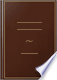Suche Bilder Maps Play YouTube News Gmail Drive Mehr »
Anmelden
 Books Bücher 21 - 30 von 36 in PROBLEM I. To find the area of a parallelogram, whether it be a square, a rectangle,...PROBLEM I. To find the area of a parallelogram, whether it be a square, a rectangle, a rhombus, or a rhomboides.An Encyclopædia of Architecture: Historical, Theoretical, and Practical - Seite 372
von Joseph Gwilt - 1842 - 1089 Seiten
Vollansicht - Über dieses Buch## A Treatise on Military Surveying: Including Sketching in the Field, Plan ...

Basil Jackson - 1847 - 344 Seiten
...is called 4 inches, and perpendicular 3 inches ; and 3x4=12 square inches. To find the area of any parallelogram, whether it be a square, a rectangle, a rhombus, or a rhomboid. Multiply the length, stated in any linear measurement, as inches, links, feet, yards, &c., by the perpendicular height,...
Vollansicht - Über dieses Buch## An Introduction to Mensuration and Practical Geometry

John Bonnycastle - 1848 - 288 Seiten
...student by observing this rule will generally find his results to agree with those given in the book. PROBLEM I. To find the area of a parallelogram ; whether it be a square, a rectangle, a rhombus, or a rhomboidfs. RULE.* Multiply the length by the perpendicular height, and the product will be the area....
Vollansicht - Über dieses Buch## Principles of Architecture: Comprising Fundamental Rules of the Art, with ...

Peter Nicholson, Joseph Gwilt - 1848 - 280 Seiten
...of the square. Hence we have the following rule for any parallelogram whatever. PROBLEM I. PLATE 50. To find the area of a parallelogram, whether it be a square, a MENSURATION. 97 Кхлмги: I. What is the area of a square ABCD, whose side AB or вс t'a 2t 3i?...
Vollansicht - Über dieses Buch## The Practical Model Calculator: For the Engineer, Mechanic, Machinist ...

Oliver Byrne - 1851 - 588 Seiten
...area or content of any figure is computed by the number of those squares contained in that figure. To find the area of a parallelogram; whether it be a square, a rectangle, a rhombus, or a rhomboides. — Multiply the length by the perpendicular height, and the product will be the area....
Vollansicht - Über dieses Buch## The Practical Model Calculator: For the Engineer, Mechanic, Machinist ...

Oliver Byrne - 1852 - 588 Seiten
...area or content of any figure is computed by the number of those squares contained in that figureTo find the area of a parallelogram; whether it be a square, a rectangle, a rhombus, or a rlwrnboides- — Multiply the length by the perpendicular height, and the product will be the areaThe...
Vollansicht - Über dieses Buch## Cyclopedia of Architecture: Historical, Descriptive, Typographical ...

Robert Stuart - 1854
...The superficial content of any figure. For example : PI M1, " Mensuration of Superficies У Prob. \. To find the area of a parallelogram : whether it be...rhombus, or a rhomboid. Multiply the length by the breadth, or perpendicular height, and the product will be the area. / ¡. 1. To find the area of a...
Vollansicht - Über dieses Buch## A complete course of questions in arithmetic & mensuration

Richard Bowling - 1858
...51H -. 84 54 27T9ff ... J^.f 55 7T\ ... .0001 56 6A ... .00001 MENSURATION OF SUPERFICIES. PROBLEM 1. To find the area of a parallelogram, whether it be a square, a rectangle, a rhombus, or a rhomboid. 1 Find the area of a -square whose side is 194 feet. 2 Find the area of a square whose side is 176...
Vollansicht - Über dieses Buch## Text-book of modern carpentry: comprising a treatise on building-timber ...

Thomas William Silloway - 1858 - 180 Seiten
...the radii; as pns, fig. 7. A quadrant is a quarter of a circle; as A6D, fig. 8. PROBLEM I. TO FIXD THE AREA OF A PARALLELOGRAM, WHETHER IT BE A SQUARE, A RECTANGLE, A RHOMBUS, OR A RHOMBOID. RULE. — Multiply the length by the perpendicular height, and the product will be the area. PROBLEM...
Vollansicht - Über dieses Buch## The Practical Model Calculator, for the Engineer, Mechanic, Machinist ...

Oliver Byrne - 1863 - 588 Seiten
...area or content of any figure is computed by the number of those squares contained in that figure. To find the area of a parallelogram; whether it be a square, a rectangle, a rhombus, or a rhomboides. — Multiply the length by the perpendicular height, and the product will be the area....
Vollansicht - Über dieses Buch## Rudimentary treatise on mensuration and measuring

1865
...square inches, or square feet, or square yards, &c. A TABLE OF SQUARE MEASURE. Acres. 1 640 Sq. Mile. 1 PROBLEM I. To find the area of a parallelogram ; whether...be a square, a rectangle, a rhombus, or a rhomboid. RULE. — Multiply the length by the breadth or perpendicular height, and the product will be the area....
Vollansicht - Über dieses Buch